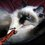# Hypergeometric distributions: A very brief introduction.

This will be a very abstract introduction, so you'll have to get used to it, and then you'll have to extend this abstraction to actual applications in the problems that are collected in this set.

The basic idea here is that we're trying to calculate the probability of getting a fixed number of "good" elements out of "bad" elements by picking a specified number of arbitrary elements. Those elements can be balls, atoms, numbers...

Let there be $n$ ways for a "good" selection and $m$ ways for a "bad" selection out of a total of $n+m$ possibilities. Take $N$ samples and let $x_i$ equal $1$ if selection $i$ is successful and $0$ if it is not. Let $x$ be the total number of successful selections,

$x\equiv\sum_{i=1}^Nx_i.$

The probability of $i$ successful selections is then

\eqalign{P(x=i) &= \dfrac{[\#\text{ ways for }i\text{ successes}][\#\text{ ways for }N-i\text{ failures}]}{[\text{total number of ways to select}]} \\ &= \dfrac{\dbinom ni\dbinom m{N-i}}{\dbinom{m+n} N}\\ &= \dfrac{m!n!N!(m+n-N)!}{i!(n-i)!(m+i-N)!(N-i)!(m+n)!}.} You will need this last formula to solve most of the problems.Note by حكيم الفيلسوف الضائع
7 years ago

This discussion board is a place to discuss our Daily Challenges and the math and science related to those challenges. Explanations are more than just a solution — they should explain the steps and thinking strategies that you used to obtain the solution. Comments should further the discussion of math and science.

When posting on Brilliant:

• Use the emojis to react to an explanation, whether you're congratulating a job well done , or just really confused .
• Ask specific questions about the challenge or the steps in somebody's explanation. Well-posed questions can add a lot to the discussion, but posting "I don't understand!" doesn't help anyone.
• Try to contribute something new to the discussion, whether it is an extension, generalization or other idea related to the challenge.

MarkdownAppears as
*italics* or _italics_ italics
**bold** or __bold__ bold
- bulleted- list
• bulleted
• list
1. numbered2. list
1. numbered
2. list
Note: you must add a full line of space before and after lists for them to show up correctly
paragraph 1paragraph 2

paragraph 1

paragraph 2

[example link](https://brilliant.org)example link
> This is a quote
This is a quote
    # I indented these lines
# 4 spaces, and now they show
# up as a code block.

print "hello world"
# I indented these lines
# 4 spaces, and now they show
# up as a code block.

print "hello world"
MathAppears as
Remember to wrap math in $$ ... $$ or $ ... $ to ensure proper formatting.
2 \times 3 $2 \times 3$
2^{34} $2^{34}$
a_{i-1} $a_{i-1}$
\frac{2}{3} $\frac{2}{3}$
\sqrt{2} $\sqrt{2}$
\sum_{i=1}^3 $\sum_{i=1}^3$
\sin \theta $\sin \theta$
\boxed{123} $\boxed{123}$